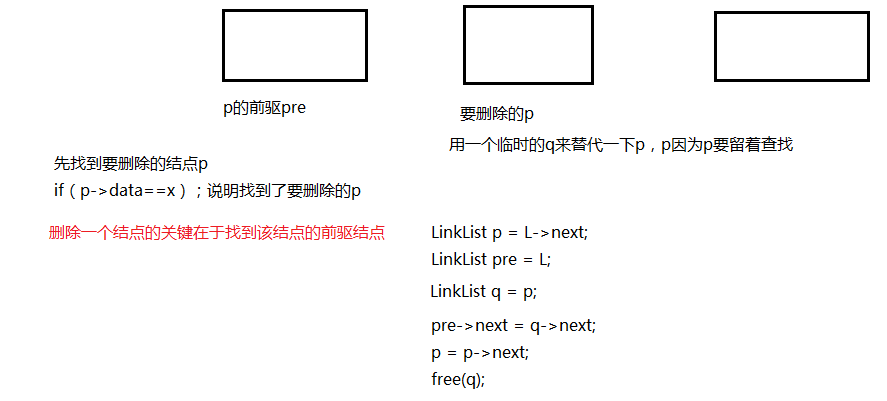#include<stdio.h>

#include<stdlib.h>

typedef struct LNode{
int data;
struct LNode *next;

p->next = NULL;
return p;
}

//带有头结点的单链表
int j = 1;//统计个数
if(i<0)
return NULL;
if(i==0)
return L;//返回头结点
while(p && j<i){
p = p->next;
j++;
}
return p;
}

//带有头结点的单链表
while(p && p->data!=e){
p = p->next;  //找不到就继续查找
}
return p;//找到就返回
}

int x;

scanf("%d",&x);
while(x!=9999){
s->data = x;
s->next = L->next;
L->next = s;
scanf("%d",&x);
}
return L;
}

int x;

scanf("%d",&x);
while(x!=9999){
s->data = x;
r->next = s;
r = s;
scanf("%d",&x);
}
r->next = NULL;
return L;

}

while(p){
printf("%d ",p->data);
p = p->next;
}
printf("\n");
}

s->data = num;
s->next = p->next;
p->next = s;

return L;

}

p->next = q->next;
free(q);

return L;
}

while(p!=NULL){
if(p->data == x){
q = p;
pre->next = q->next;
p = p->next;
free(q);

}
else{
pre = p;//关键pre要一直指向p的前驱
p = p->next;
}
}
return L;
}

int main(){

int x = 1;
int y = 0;
int num = 0;
scanf("%d%d",&x,&num);
while(x!=99 && num!=99){
Insert_Elem(L,x,num);
scanf("%d%d",&x,&num);
}
ListTraverse(L);

scanf("%d",&y);
Delete_SameX(L,y);
ListTraverse(L);
return 0;
}

展开全文• 如何删除单链表中第i个节点？ 先来看看删除的原理：因为数据结构是单链表，要想删除第i个节点，就要找到第i个节点；要想找到第i个节点，就要找到第i-1个节点；要想找到第i-1个节点，就要找到第i-2个节点......于是...
如何删除单链表中第i个节点？

先来看看删除的原理：因为数据结构是单链表，要想删除第i个节点，就要找到第i个节点；要想找到第i个节点，就要找到第i-1个节点；要想找到第i-1个节点，就要找到第i-2个节点......于是就要从第一个节点开始找起，一直找到第i-1个节点。如何找？让一个指针从头结点开始移动，一直移动到第i-1个节点为止。这个过程中可以用一个变量j从0开始计数，一直自增到i-1。

之后呢？我们把第i-1个节点找到了，就让它的指针域指向第i+1个节点，这样就达到了删除的目的。而第i+1个节点的地址又从第i个节点获得，第i个节点的地址又是第i-1个节点的后继。因此我们可以这样做：先让一个指针指向第i-1个节点的后继，（保存i+1节点的地址），再让i-1节点的后继指向第i个节点的后继，这样就将第i个节点删除了。

再来看看删除的时候会遇到什么意外情况：

1.有可能单链表一开始就为空。这样的话连第i-1个元素都找不到。

2.有可能找不到第i个节点，原因是第i-1的后继为空。

3.有可能删除的位置不合理，比如删除第-1个节点。

如何删除单链表中数据域为x的前驱节点？

这个删除操作其实和上面的类似。关键是要知道三个地址，p->第i-2个节点的地址，q->第i-1个节点的地址，r->元素为x的第i个节点的地址。（为什么？因为我们要删除的是第i-1个节点。要想删除它，就既要找到第i-2个节点，又要找到元素为x的第i个节点）

假设三个指针，p，q，r。p=L（L为头结点的地址）。q=p->next；（这里要注意先判断q是否为空？如果q为空，意味着L->next为空。空链表，不予受理！！！！！！）
r=q->next;(这样子，三个指针就连续了)

当r->data!=X,并且r！=null时（最前面的指针指向的节点数据域不是x，并且这个指针有节点去指向）

让指针移动，p=q；q=r；r=r->next;这样就找到了满足条件的三个地址。

找到了地址，下一步是删除。要注意的一点是，什么时候可以删除?只有当r!=null，才能删。否则不满足题目一开始的条件了。

删除操作：p->next=q->next;free(q);

展开全文• cout输入查找值："; cin>>num; int find_data=FindData(p,num); if(find_data!=ERROR) { cout查找结果："; } else { cout无法查询结果"; } } } return 0; }
#include<iostream>
#include<stdlib.h>
#define ERROR -40000
using namespace std;

typedef struct LNODE
{
int data;
struct LNODE *next;
}Lnode;

Lnode *Input(int n)
{
p->next=NULL;
for(int i=0;i<n;i++)
{
int data;
cin>>data;
q=(Lnode*)malloc(sizeof(Lnode));
q->data=data;
q->next=p->next;
p->next=q;
p=q;
}
}

int FindData(Lnode *L,int num)
{
Lnode *p;
p=L->next;
int id=1;
while(p!=NULL)
{
if(p->data==num) return id;
id++;
p=p->next;
}
if(p==NULL) return ERROR;
}

int main()
{
int n;
while(cin>>n)
{
Lnode *p=Input(n);
cout<<"数据查询："<<endl;
while(1)
{
int num;
cout<<"输入查找值："<<endl;
cin>>num;
int find_data=FindData(p,num);
if(find_data!=ERROR)
{
cout<<"查找结果为："<<find_data<<endl;
}
else
{
cout<<"无法查询结果"<<endl;
}
}
}
return 0;
}

展开全文• 单链表查找最大并删除 任务描述： 试编写带头结点的单链表删除一个最大结点的高效算法。 代码实现： // 带头节点的单链表删除一个最大结点 #include <stdio.h> #include <stdlib.h> ...
单链表查找最大值并删除
任务描述：
试编写在带头结点的单链表中删除一个最大值结点的高效算法。
代码实现：
// 在带头节点的单链表中删除一个最大值结点

#include <stdio.h>
#include <stdlib.h>

using namespace std;

// 单链表结构
typedef struct LNode{
int data;
struct LNode *next;

// 建立单链表（头插法）
int x;
L = (LNode*)malloc(sizeof(LNode)); //创建头结点
L->next = NULL;
for(int i=0;i<n;i++){
scanf("%d",&x);
S = (LNode*)malloc(sizeof(struct LNode));
S->data = x;
S->next = L->next;
L->next = S;
}
return L;
}

// 建立单链表（尾插法）
int x;
L = (LNode*)malloc(sizeof(LNode));
LNode *S,*T = L;
for(int j=0;j<n;++j){
scanf("%d",&x);
S = (LNode*)malloc(sizeof(LNode));
S->data = x;
T->next = S;
T = S;
}
T->next = NULL;
return L;
}

// 输出单链表
p = L->next;
printf("当前链表元素为：\n");
while(p != NULL){
printf("%d ",p->data);
p = p->next;
}
}

// 寻找单链表最大值的位置
int temp; //利用temp标注最大值位置
int MAX;
S = L->next;
MAX = S->data;
temp = 0;
for(int i=0;i<n;i++){
if(S->data > MAX){
MAX = S->data;
temp = i;
}else{
MAX = MAX;
}
S = S->next;
}
return temp;
}

// 删除链表中元素
int j = 0;
while(S->next&&j<i){
S = S->next;
j++;
}
T = S->next;
S->next = T->next;
*e = T->data;
free(T);
return 0;
}

int main(){
int n; // 链表元素个数
int MAX_value; //链表最大值
printf("请输入链表元素个数：\n");
scanf("%d",&n);
printf("输入链表中元素：\n");
L = CreateList_Tail(L,n);
PrintList(L);
putchar('\n');
printf("当前链表最大值为：\n%d",MAX_value);
putchar('\n');
PrintList(L);
return 0;
}

结果示例：展开全文链表
• C语言查找某数据在单链表中出现的次数 ...2.链表数据元素；3.待查找的元素 【输出形式】待查找元素出现的次数 【样例输入】 5 521 3 9 48 6 9 【样例输出】1 #include<stdio.h> #include<stdlib.h> ...c语言
• 在查找过程，设置两个指针，让其中一个指针比另一个指针先前移k步，然后两个指针同时往前移动。循环，直到先行的指针值为NULL时，另一个指针所指的位置就是所要找的位置 struct ListNode { int val; ListNode *...指针 算法 链表
• 本题要求实现一个函数，可统计带头结点的单链表中大于指定元素个数。 函数接口定义： int GetLarger( LinkList L,ElemType e ); L是带头结点的单链表的头指针，e是要比较的元素值。函数GetUp返回单链表中大于e...
• p指针用来找链表数字为x的位置，pre指针始终指向p指针所指向位置的前一个位置 最好自己纸上模拟一下 代码： #include&lt;bits/stdc++.h&gt; using namespace std; typedef struct Node { int value...
• 获取表L第i个位置的元素。//按位查找，返回第i个元素(带头结点)LNode * GetElem(LinkList L, int i){if(i<0)return NULL;LNode *p;//指针p指向当前扫描到的结点int j=0;//当前p指向的是第几个结点p = L;//L...
• 单链表元素查找并插入，若不存在需要查找元素，则在单链表最后插入 代码实现 #include <stdio.h> #include <stdlib.h> #include <malloc.h> #define FLAG -1 //停止输入的标志 typedef struct...线性表
• bool DeListFirstElem(LinkList &L,ElemType x){ LNode *pre=L; LNode *p=pre->...=NULL){//找值为x的结点，找不到pre和p都后移一位 pre=p; p=p->next; } if(p!=NULL){//找到p pre->next=
• 单链表查找操作 一. 按序号查找 #include<iostream> using namespace std; typedef struct LNode { int data; struct LNode *next; } *LinkList,LNode; // 尾插建立单链表 LinkList List_ToolInsert ...
• 具有链接存储结构的线性表，它用一组地址任意的存储单元存放线性表数据元素，逻辑上相邻的元素物理上不要求也相邻，不能随机存取。一般用结点描述：结点（表示数据元素） =数据域（数据元素的映象） + 指针域...链表 指针
• 查找操作 按序号查找 按指定的值查找 按序号查找单链表的第一个元素结点起， 判断当前结点是否是第i个，若是，则返回该结点的指针，...{ //在单链表L中查找第i个元素结点 LNode *p; int j; p=L->next; //
• 单链表查找–带头结点 按位查找值查找 按位查找 /** * 按位查找，返回第i个节点 * 带头结点 * @param L * @param i * @return */ LNode * GetElem(LinkList L, int i){ if (i<0){ return NULL;...
• 查找序号是X元素 注：创建单链表的时候使用一个数组初始化，避免不停的手动输入（太麻烦） 注：查找算法的错误处理什么的不健全，大家根据需要修改 代码#include using namespace std;typedef int elemtype...单链表
• 单链表的存储结构： typedef struct LinkList{ int data; LinkList * next; } 分析： 如果我们用一个指针p ，指向要删除的结点， 要删除第一个还好，可以删， 如果光一个指针p中间你怎么删？ 所以，这里我们...指针 链表链表
• GetElem(L,i):按位查找，获取表L第i个位置的元素 LNode * GetElem(LinkLIst L,int i){ if(i<0) return NULL; LNode *p;//指针p指向当前扫描到的结点 int j=0;//当前p指向的是第几个结点 p=L;//L指向头...链表
• 首先，我们要删除第一个值为x结点的前驱结点，则该链表要包含两个及以上结点才行。 前面通常都是删除该节点，而这次是删除该节点的前驱结点。 所以不能向以往一样定义两个指针。而应该定义三个指针。 prepre , pre...指针 链表
• 可供操作 printf("\n输入你要选择的... printf("5、查找信息\n"); printf("6、删除信息\n"); printf("7、退出\n"); 代码使用了后插入法 #define _CRT_SECURE_NO_WARNINGS #include "stdio.h" #include "s.
• 1. 按位查找(平均时间复杂度O(n)) GetElem(LinkList L, int i) 返回第i个元素 LNode * GetElem(LinkList L, int i){ if(i<0) return NULL; LNode *P; p = L; int j = 0; //当前p指向的第几个节点 while...
• 的方式删除单链表中的所有值为x的结点，如果是普通的线性表删除，相信大家都会做，但是不带头结点的情况以递归的方式删除就比较烧脑。 由于最近时间比较近，来不及画图描述我的思想，我们直接研究答案吧！ 上我写...
• 设计一个算法带头结点的非空单链表L第一个最大节点（最大节点可能有多个）之前插入一个值为x的结点 使用maxpos 记录第一个最大节点所出现的位置， 之后进行简单的单链表插入即可。 代码如下： #...算法 链表
• 给若干个数字，默认int类型，要求使用单链表存放，现需要寻找该单链表中位于最中间的数字，要求使用一种时间和空间上都高效的算法实现。 思想： 单链表是一种线性结构，每个元素只可有他的前驱结点进行访问，若...
• 本题目摘自《Python程序员面试算法宝典》，我会每天做一道这本书上的题目，并分享出来，统一放在我博客内，收集一个分类。 【微软笔试题】 ... 7，则单链表的倒数第3个元素为5. 方法一：顺序遍历法 这种...python 算法...